# Geometry Symbols

## Table of symbols in geometry:

Symbol Symbol Name Meaning / definition Example
angle formed by two rays ∠ABC = 30°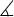measured angleABC = 30°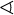spherical angleAOB = 30°
right angle = 90° α = 90°
° degree 1 turn = 360° α = 60°
deg degree 1 turn = 360deg α = 60deg
prime arcminute, 1° = 60′ α = 60°59′
double prime arcsecond, 1′ = 60″ α = 60°59′59″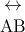line infinite line
AB line segment line from point A to point B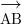ray line that start from point A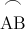arc arc from point A to point B= 60°
perpendicular perpendicular lines (90° angle) ACBC
parallel parallel lines ABCD
congruent to equivalence of geometric shapes and size ∆ABC ≅ ∆XYZ
~ similarity same shapes, not same size ∆ABC ~ ∆XYZ
Δ triangle triangle shape ΔABC ≅ ΔBCD
|x-y| distance distance between points x and y | x-y | = 5
π pi constant π = 3.141592654...

is the ratio between the circumference and diameter of a circle

c = πd = 2⋅πr

Algebra symbols ►